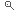PORTALE DELLA DIDATTICACERCA KEYWORD

#### Symbolic regression using Robust Evolutionary Polynomial Regression for data noise compensation in engineering

keywords EVOLUTIONARY ALGORITHMS

Reference persons GIUSEPPE CARLO MARANO

Thesis type EXPERIMENTAL AND SIMULATION

Description Machine learning (ML) is a subset of artificial intelligence that should commonly be defined as the study of algorithms and statistical models that computer systems use to perform a specific task without explicit instructions, but relying on patterns and inference instead. Its algorithms build a mathematical model based on sample data, known as "training data", in order to make predictions or decisions without being explicitly programmed to perform the task. ML algorithms are used in a wide variety of applications, such as email filtering and computer vision, where it is difficult or infeasible to develop a conventional algorithm for effectively performing the task. In the field of Machine learning, symbolic regression (SR) is a type of regression analysis that searches the space of mathematical expressions to find the model that best fits a given dataset, both in terms of accuracy and simplicity. Without a pre-specified model structure, it is challenging to fit symbolic regression, which requires to search for the optimal solution in a large space of mathematical expressions and estimate the corresponding parameters simultaneously. Instead, initial expressions are formed by randomly combining mathematical building blocks such as mathematical operators, analytic functions, constants, and state variables. (Usually, a subset of these primitives will be specified by the person operating it, but that's not a requirement of the technique.)
Traditionally, symbolic regression is solved by combinatorial optimization methods like Genetic Programming (GP) that evolves over generations. However, GP suffers from high computational complexity and overly complicated output expressions, and the solution is sensitive to the initial value.
By not requiring a specific model to be specified, symbolic regression isn't affected by human bias, or unknown gaps in domain knowledge. It attempts to uncover the intrinsic relationships of the dataset, by letting the patterns in the data itself reveal the appropriate models, rather than imposing a model structure that is deemed mathematically tractable from a human perspective. The fitness function that drives the evolution of the models takes into account not only error metrics (to ensure the models accurately predict the data), but also special complexity measures, thus ensuring that the resulting models reveal the data's underlying structure in a way that's understandable from a human perspective. This facilitates reasoning and favors the odds of getting insights about the data-generating system.

Deadline 29/07/2021      PROPONI LA TUA CANDIDATURA